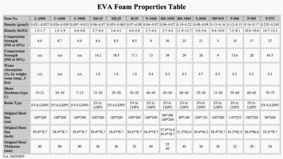### TEAR RESISTANCE

Tear Resistance is the force required to rip a sample of plastic film, sheeting or rubber product. An unnicked 90-degree angle specimen is used to measure the tear resistance of foam.
Calculation:

Calculate the resistance to tear from the maximum force registered by the testing machine and the thickness of the specimen, and express as the force in newtons (or kilogram, pounds-force) required to tear a specimen 1 m (or 1cm, 1 inch) in thickness.

Tear Resistance = F / t

where:
F = force, N (kg, lbf).
t = specimen thickness, m (cm, inch).More technical data of EVA foam material